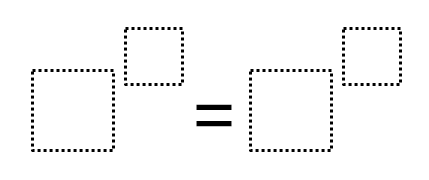# Equivalent Exponents

Directions: Using the digits 0-9 only once each, create as many true equations as possible.### Hint

How can you use the fact that anything to the zero power equals one?
In what ways are 3 and 9 are connected, as are 2, 4 and 8?
How can we use this knowledge to build on creating equivalent expressions?

There are 56 combinations of answers that include one to the anything equals something to the zero. Aside from that, I have found
8^1=2^3
9^1=3^2
8^2=4^3
9^2=3^4
8^3=2^9
9^4=3^8
8^6=4^9

Source: Annie DeAngelo and Maeve O’Connell

## Solving One-Step Inequalities with Addition

Directions: Using the digits 0 to 9 at most one time each, place a digit …

1.Some examples are 8^3=2^9,8^6=4^9,8^1=2^3, and 8^2=4^3.

2.8^1=2^3
8^2=4^4
8^3=2^9
8^6=4^9

3.Porque seria el mismo dijito sumado porque como si lo miras biennes el mismo dijito porque algunos son ejemplos

4.Danitza Marenco

8^1=2^3
8^2=4^4
8^3=2^9
8^6=4^9

5.8 to the power of 2=4 to the power of 3

6.8^2 = 4^3
3^2 = 9^1

7.8^1=2^3
9^1=3^2
8^2=4^3
9^2=3^4
8^3=2^9
9^4=3^8
8^6=4^9